﻿ 岩石孔径分布特征对其力学性质的影响规律研究

岩石孔径分布特征对其力学性质的影响规律研究Research on the Influence Law of the Pore Size Distribution of Rock on Its Mechanical Properties

Abstract: Rock is a natural porous material. Its pore structure will change its physical and mechanical properties, thus affecting the stability of the overall structure of the rock. Rocks with different porosity have different mechanical properties. The rocks with the same porosity and different distribution characteristics of pore size have different mechanical properties. Therefore, this paper focuses on the influence of porosity and pore size distribution on rock mechanical properties under uniaxial compression. The main contents are as follows: 1) Based on the mercury injection test results of the granite pore size distribution structure at different temperatures, numerical value models were established for nine granite samples with porosity of 2%, 5%, and 10%, and pore size distributions of 2, 2.5 and 3 fractal dimensions. 2) The differences in rock mechanical properties under different pore size distributions were studied, and the influence laws of the fractal dimension of porosity and pore size distribution on the stress-strain curve, compression strength, elastic modulus, crack propagation path and failure mode of the rock were obtained.

1. 引言

1) 孔隙率对岩石应力–应变曲线、抗压强度、弹性模量、破坏方式的影响。

2) 孔隙孔径分布分形维数对岩石应力–应变曲线、抗压强度、弹性模量、破坏方式的影响。

2. 数值试验概述

2.1. 材料参数

2.2. 孔洞半径Table 1. System resulting data of standard experimentTable 2. The radius of each circular hole when the fractal dimension is 2Table 3. The radius of each circular hole when the fractal dimension is 2.5

$D=\frac{L{g}^{N}}{L{g}^{r}}$

2.3. 数值试验方法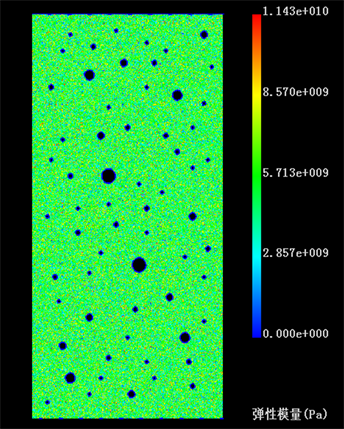Figure 1. The 2D model of the pore when the porosity is 2% and the fractal dimension is 2Table 4. The radius of each circular hole when the fractal dimension is 3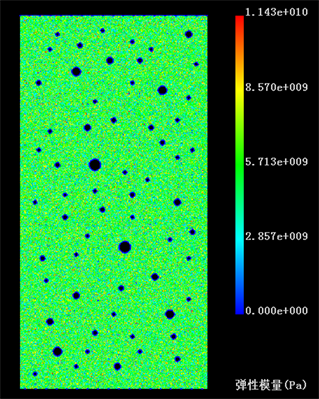Figure 2. The 2D model of the pore when the porosity is 2% and the fractal dimension is 2.5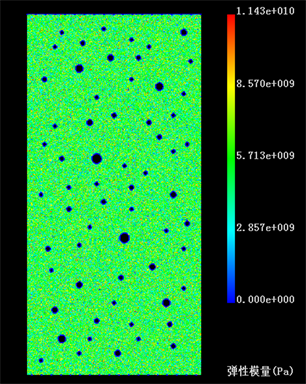Figure 3. The 2D model of the pore when the porosity is 2% and the fractal dimension is 3

3. 数值试验结果分析

3.1. 破坏过程分析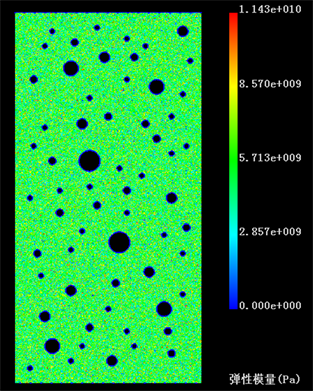Figure 4. The 2D model of the pore when the porosity is 5% and the fractal dimension is 2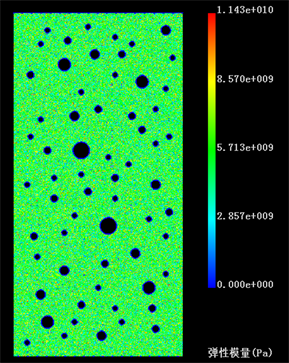Figure 5. The 2D model of the pore when the porosity is 5% and the fractal dimension is 2.5

1) 在相同分形维数情况下，岩石试样随着孔隙率的增加破坏的速度越来越快，即孔隙率越大完全破坏时所需加载的步数越少。

2) 几乎所有模型都是先贯通最大的两个孔洞，并最终大致沿着两个孔洞圆心连成的直线方向与上下边界贯通，只有在孔隙率 = 2，分形维数D = 3的时候破环方式不同，可能是由于孔隙率太小并且分形维数有点大导致各个圆孔的大小差别不是太明显引起的原因。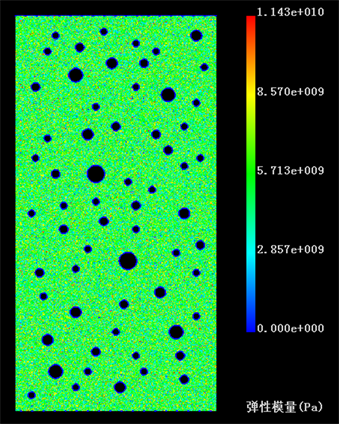Figure 6. The 2D model of the pore when the porosity is 5% and the fractal dimension is 3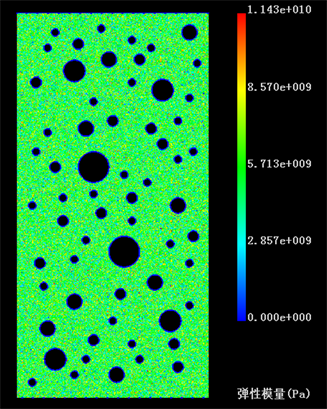Figure 7. The 2D model of the pore when the porosity is 10% and the fractal dimension is 2

3) 所有模型的破坏方式都是拉伸破坏和剪切破坏相结合。

4) 在同一孔隙率下，分形维数D越大在完全破坏时明显的裂纹越多。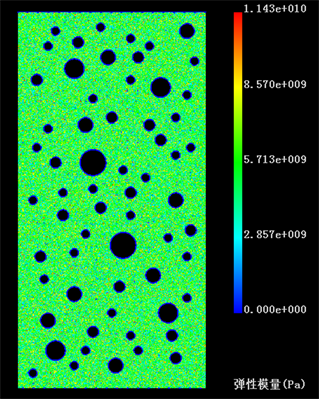Figure 8. The 2D model of the pore when the porosity is 10% and the fractal dimension is 2.5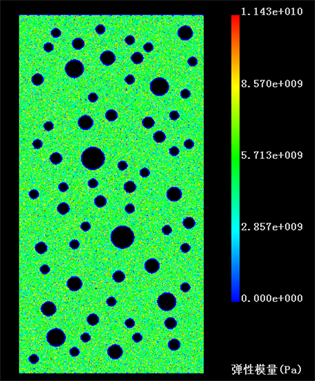Figure 9. The 2D model of the pore when the porosity is 10% and the fractal dimension is 3

3.2. 孔隙率对岩石应力–应变曲线的影响分析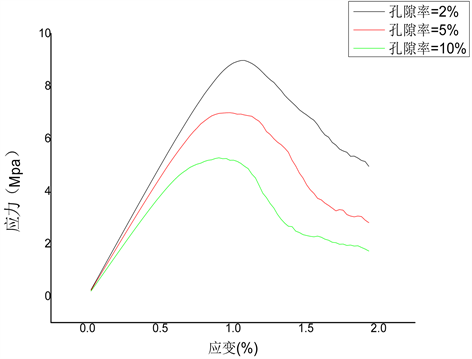Figure 10. Stress-strain diagram when fractal dimension D = 2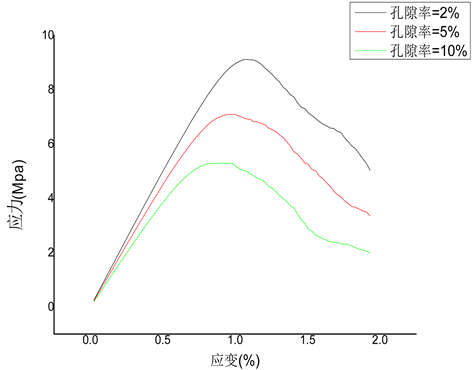Figure 11. Stress-strain diagram when fractal dimension D = 2.5

1) 在同一种分形维数情况下，峰值应力随着孔隙率的增大而减小，孔隙率为2%时峰值应力最大，孔隙率为10%时最小，且差距比较大。

2) 上面三组岩石模型在达到峰值应力前的大部分时间都呈线性变化，处于弹性变形阶段；且应力增长的速度随着孔隙率的变化而改变，孔隙率越小应力增长速度越快。

3) 在同一种分形维数情况下，孔隙率越大达到峰值应力所需的加载步数越少，在本次实验中，孔隙率为10%时所需的步数最少，孔隙率为2%时所需的步数最多。

3.3. 孔隙分形维数对应力–应变曲线的影响分析

1) 在同一孔隙率情况下，峰值应力随着分形维数D的增加而增加，在分形维数D为2时峰值应力最小，在分形维数D为3时最大，但是差距不是太大。

2) 上面三组岩石模型在达到峰值应力前的大部分时间都呈线性变化，处于弹性变形阶段；且通过对曲线斜率的计算知道应力增加的速度随着分形维数D的增加都加快，但变化不明显。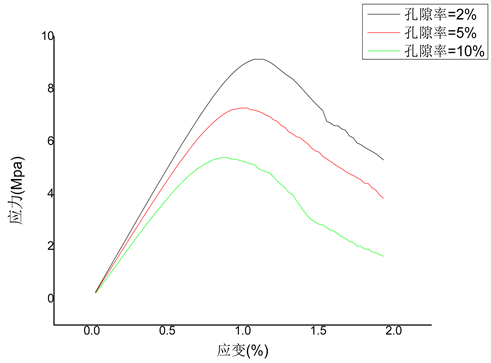Figure 12. Stress-strain diagram when fractal dimension D = 3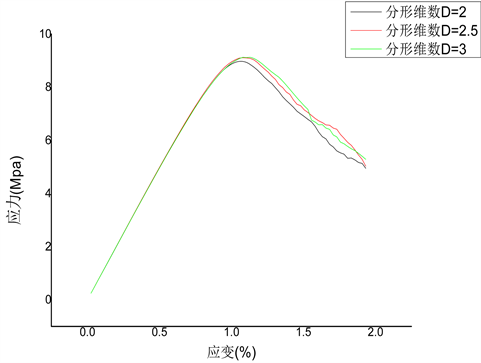Figure 13. Stress-strain diagram when porosity = 2%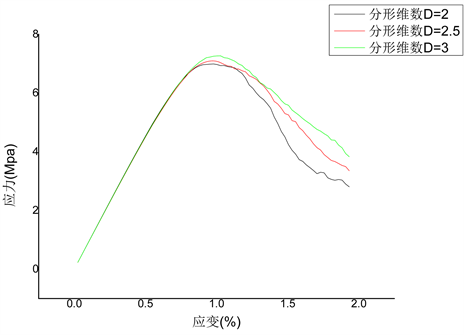Figure 14. Stress-strain diagram when porosity = 5%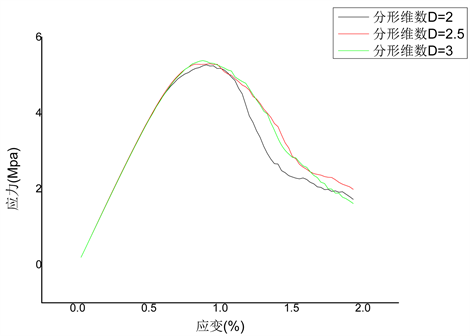Figure 15. Stress-strain diagram when porosity = 10%

3.4. 孔隙率和孔隙分形维数对抗压强度的影响分析

1) 在同一分形维数情况下，抗压强度的大小随着孔隙率的变化而发生变化，孔隙率越小抗压强度越大，在本次实验中，孔隙率为2%时抗压强度最大，孔隙率为10%时抗压强度最小，且相互之间差距比较大。

2) 在相同孔隙率的情况下，抗压强度的大小随着分形维数的变化也发生变化，分形维数D越大时抗压强度越大，在这次实验中，分形维数D等于3时抗压强度最大，分形维数D等于2时抗压强度最小，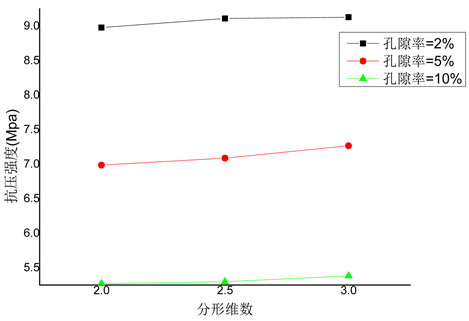Figure 16. The relationship between porosity and compressive strength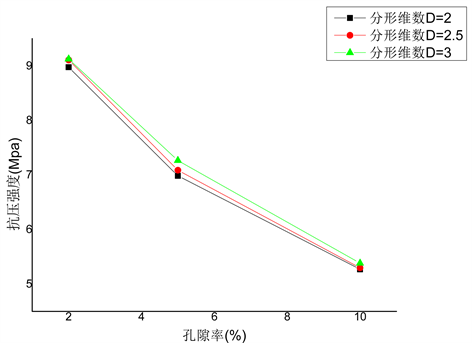Figure 17. The relationship between fractal dimension and compressive strength

3) 抗压强度的差值随着孔隙率间差距的变大而变小。

4) 在孔隙率为2%时，分形维数D等于2.5和分形维数D等于3情况下抗压强度特别接近，在孔隙率为10%时，分形维数D等于2和分形维数D等于2.5情况下抗压强度接近，在孔隙率等于5%时抗压强度在不同分形维数情况下有一定的差距。

3.5. 孔隙率和孔隙分形维数对弹性模量的影响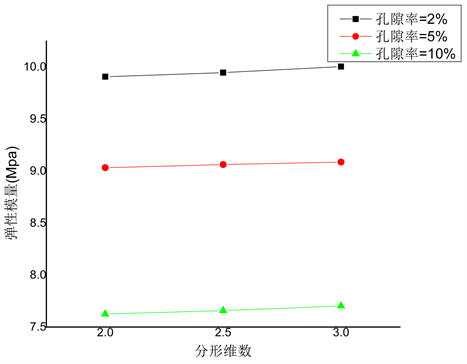Figure 18. The relationship between porosity and elastic modulus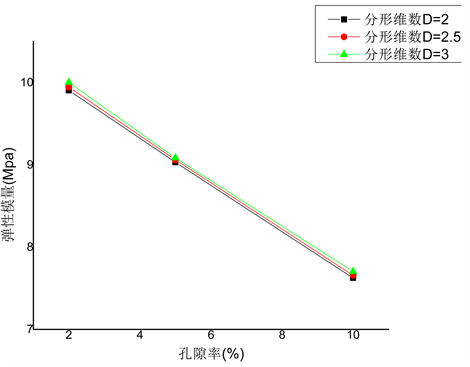Figure 19. The relationship between fractal dimension and elastic modulus

1) 在同一分形维数情况下，弹性模量的大小随着孔隙率的变化而发生变化，孔隙率越小弹性模量越大，在本次实验中，孔隙率为2%时弹性模量最大，孔隙率为10%时弹性模量最小，且相互之间差距比较大。

2) 在相同孔隙率的情况下，弹性模量的大小随着分形维数的变化也发生变化，分形维数D越大时弹性模量越大，在这次实验中，分形维数D等于3时弹性模量最大，分形维数D等于2时抗压强度最小，但相互之间差距不太明显。

4. 结论与展望

1) 在同一分形维数情况下，岩石试样随着孔隙率的增加破坏的速度越来越快,即孔隙率越大完全破坏时所需加载的步数越少；在弹性阶段应力增长的速度随着孔隙率的减小而变快，但是达到峰值应力所需的加载步数却随着孔隙率的减小而增多；孔隙率越小，岩石的抗压强度和弹性模量就越大，且差距比较明显。

2) 在同一孔隙率情况下，分形维数D越大岩石模型在完全破坏时明显的裂纹就越多；在弹性阶段应力增加的速度随着分形维数D的增加都变快，但不明显；分形维数D越大，岩石模型的抗压强度和弹性模量也越大，但差距较小。

3) 本文中全部选用摩尔库伦破坏准则，而没有将其与其它的Hoek-Brown准则、Double-shear准则和Single-shear准则进行对比，探讨各种准则的适用范围。

NOTES

*通讯作者。

 Harrison, J.P. and Hudson, J.A. (2000) Engineering Rock Mechanics: An Introduction to the Principles. Elsevier Science Ltd., Ne-therland, 5-20.

 杨永明, 鞠杨, 刘红彬, 等. 孔隙结构特征及其对岩石力学性能的影响[J]. 岩石力学与工程学报, 2009, 28(10): 2031-2038.

 张志镇, 高峰, 高亚楠, 等. 高温影响下花岗岩孔径分布的分形结构及模型[J]. 岩石力学与工程学报, 2016, 35(12): 2426-2437.

 马新仿, 张士诚, 郎兆新. 分形理论在岩石孔隙结构研究中的应用[J]. 岩石力学与工程学报, 2003, 22(增1): 2164-2167.

 杨庆红, 谭吕, 蔡建超, 等. 储层微观非均质性定量表征的分形模型[J]. 地球物理学进展, 2012, 27(2): 603-609.

 Lai, J. and Wang, G.W. (2015) Fractal Analysis of Tight gas Sandstones Using High-Pressure Mercury Intrusion Techniques. Journal of Natural Gas Science and Engineering, 24, 185-196. https://doi.org/10.1016/j.jngse.2015.03.027

 Giri, A., Tarafdar, S., Gouze, P., et al. (2015) Multifractal Analysis of the Pore Space of Real and Simulated Sedimentary Rocks. Geophysical Journal International, 200, 1106-1115.

 Zhang, Z.Y. and Weller, A. (2014) Fractal Dimension of Pore-Space Geometry of an Eocene Sandstone Formation. Geophysics, 79, D377-D387. https://doi.org/10.1190/geo2014-0143.1

 朱万成, 唐春安, 等. 岩石破裂过程分析RFPA2D系统的细观单元本构关系及验证[J]. 岩石力学与工程学报2003, 22(1): 2429-2435.

 唐春安, 赵文. 岩石破裂全过程分析软件系统RFPA2D [J]. 岩石力学与工程学报, 1997, 16(5): 507-508.

Top